Ask your WordPress questions! Pay money and get answers fast!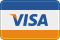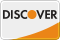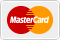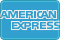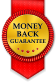# Simple math in PHP WordPress

• SOLVED

I have a variable that holds a number:

\$price

If I echo that variable, it outputs the number. In my testing, this variable equals 4995.

I simply want to get 20% of that number and echo it.

Here's my code:

``` \$DownPayment = \$price * .3; //\$price equals 4995 echo \$DownPayment; //outputs "1.2". Should be "1498.5"... WTF!! ```

If I replace the \$price variable with a hard coded number then it works. I also tried using (int)\$price, that that didn't make a difference.

I've spent 2 hours on this, and I can't figure out such a simple thing!

2011-06-22

Add this before the code, and let me know the output

`var_dump(\$price);`

Thanks for the fast reply! I get this:

string(5) "4,995"

You need to remove the "," from it first.

```\$price = str_replace(",", "", \$price); \$DownPayment = \$price * .3; //\$price equals 4995 echo \$DownPayment;```

And just to be more clear, you can do to ensure that price is an integer.

`\$price = intval(str_replace(",", "", \$price));`

That's it!!! Thank you so much!! :D

2011-06-22

Hi,

<blockquote>I simply want to get 20% of that number and echo it.</blockquote>

I thought 20% should be multiply by 0.2 and not 0.3?

if price is 4995, which is 100%
20% should be 4995 divide by 100 multiply by 20 and the answer should be 999.

Thanks.

2011-06-22

``` <?php \$price = '4,995'; \$bad_symbols = array(",", "."); \$price = str_replace(\$bad_symbols, "", \$price); \$DownPayment = \$price * .3; //\$price equals 4995 echo \$DownPayment; ?> ```